﻿ Experimental Research of Heat Transfer in Cooling Tower

### Experimental Research of Heat Transfer in Cooling Tower

I. M. KuzmenkoOPEN ACCESSPEER-REVIEWED

## Experimental Research of Heat Transfer in Cooling Tower

I. M. KuzmenkoNTUU “Kyiv polytechnic institute”, Kyiv, Ukraine

### Abstract

Heat transfer coefficient for cooling tower is determined experimentally and theoretically, the latent heat and air flow heat to are calculated. Thickness of heat layer is calculated and is then compared to theoretically calculated the displacement layer. The heat transfer coefficient is calculated based on the values of displacement layer and its deviation from theoretically calculated one is up to 3 times in the range of air velocity of 1.3-3.0 m/s. The difference in temperature of the air and water film is calculated.

### At a glance: Figures

1234
Prev Next

• Kuzmenko, I. M.. "Experimental Research of Heat Transfer in Cooling Tower." American Journal of Energy Research 2.3 (2014): 47-52.
• Kuzmenko, I. M. (2014). Experimental Research of Heat Transfer in Cooling Tower. American Journal of Energy Research, 2(3), 47-52.
• Kuzmenko, I. M.. "Experimental Research of Heat Transfer in Cooling Tower." American Journal of Energy Research 2, no. 3 (2014): 47-52.

 Import into BibTeX Import into EndNote Import into RefMan Import into RefWorks

### 1. Introduction

The heat and mass transfer processes in cooling towers require calculation of the evaporative cooling of water. These depend on the water and air velocities, contact area and the input temperatures.

There are four types of models for calculating of the heat and mass transfer processes at evaporative cooling.

The first group is based on the liquid heat balance equation with substitution of Lewis number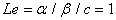(Merkel’s equation) [1, 2, 3]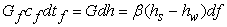(1)

Equation (1) illustrates the temperature of water film depend on mass transfer between air and water film and gives good results at wet-bulb water temperature (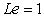).

The next type of models is based on the calculation of heat flow to water film by heat and mass flow [4, 5, 6]. Next equations describe evaporative cooling of water.

Heat balance of water film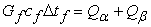(2)

where heat flow at cooling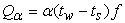(3)

and mass flow at evaporation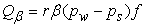(4)

The model uses the known values of heat and mass transfer coefficients and gives the output temperatures of the component, partial pressure of vapour.

Another type of models uses system of differential equations describes the evaporative cooling of water film at countercurrent flow [7, 8].

Energy equation for water film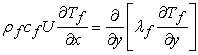(5)

Energy equation for air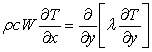(6)

Concentration in air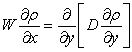(7)

and the heat balance equation (2).

After specifying the boundary conditions, system (5-7) is solved numerically.

The model (5-7) was modified by reducing of the water film thickness [9, 10]. But this increased the deviation of theoretical and experimental result.

Another group of models is based on the numerical study of system continuity, momentum, energy and concentration equations for air and water film [11, 12, 13, 14] at concurrent water-air flow. This group of models allows calculating values of the heat and mass transfer coefficients, but deviation between numerical and experimental results for this group of models is up to 33%.

Models mentioned above, need experimental determination of the heat mass transfer coefficients that limits their application, existence of the Lewis analogy determines the accuracy of output temperatures and partial pressure drop.

So, the aim of this paper is (i) to calculate theoretically heat transfer at evaporative cooling using theory of boundary layer in air and water film, (ii) to study experimentally the heat transfer characteristics and pressure drop of the cooling tower, (iii) to check the deviation between the experimental and theoretical result.

### 2. Theoretical Features of Heat and Mass Transfer in Dry Air

Calculation of contact devices is based on the inherent processes analysis. Let us consider these processes. The initial parameters of water and air determine the heat and mass transfer in the contact apparatus. The processes of heat and mass transfer in the contact device are described on humidity diagram (Figure 1).

Point O correspond to initial state of the air with dry-bulb tc, wet-bulb temperature tm, enthalpy h0 and absolute humidity d0. Air contacts with water of different initial temperature (point 1-7 in Figure 1), or more precisely – with different air temperature close to water. The interaction of air and water may be as follows:

1. Polytropic air cooling and water subtracting with its heating;

2. Air cooling at dew point with water heating;

3. Polytropic saturation and air cooling with water heating;

4. Adiabatic saturation and air cooling without changing water temperature;

5. Polytropic saturation and cooling the air with cooling water;

6. Isothermal saturation of the air with water cooling;

7. Polytropic air saturation and heating with water cooling.

It should be noted that the processes of: air cooling at dew point with water heating, adiabatic saturation and air cooling without changing water temperature, isothermal saturation of the air with water cooling are not used in engineering applications. Therefore, we will further study processes of air saturation which can take place in cooling towers of different types (labeled D and J in Figure 1).

### 3. Experimental Unit and Results

The processes of air humidification in the cooling tower mode with cooling down hot water by means of air  are shown at Figure 2.

Figure 2. Structure of the experimental unit (1, 3 – rotameters, 2, 4 – electrical heaters, 5 – experimental area, 6 – thermocouples)

The unit worked as follows. Air and water with certain mass flow and temperatures are fed to the experimental area which is a model of cooling tower with corrugated netting packing and countercurrent flow shown at Figure 3. Movement of heat transfer agents is reverse. Absolute moisture content is calculated from the heat balance.

As a result of the unit testing the range of the heat transfer agents is established, heat losses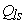in the mode of work without area with corrugated netting packing for all the air velocities are evaluated.

The experiment was conducted according to the standard plan and consisted of the air loss variation at the stable input water and air temperatures.

Main operating and geometrical parameters are specified in Table 1.

As a result of this the mass transfer coefficient value is established using the technique mentioned above.

#### Table 1. Main Parameters

Knowing the input parameters of water and air, area of the contact device and final parameters of the heat transfer agents we can determine the mass transfer coefficient in the contact device.

If the water temperature is higher than the air temperature due to the difference of temperatures the water is heated and moisturized because of the difference of partial pressures.

The research data obtained includes losses of water and air at the output (measured with the rotameters), temperatures of water and air at the input and output (measured with the dry thermocouples). Sometimes the thermocouple at the output was wetted and therefore it screened. The results of experiment are specified in Table 2.

The research data is processed according to the following pattern.

#### Table 2. The Results of Experiment

Output relative humidity which depends on velocity is calculated. So the amount of the water evaporated was determined from the heat balance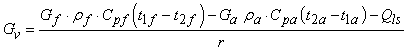(8)

where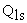are the heat losses.

The output absolute humidity, output steam pressure, maximum steam pressure in the air at the output air temperature is calculated as follows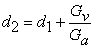(9)

where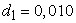,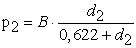,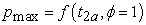. Relative humidity is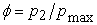.

Further the correlation of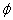from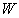was checked. Type of correlation dependence is selected based on the available results and the data which was reducing the R – Pearson’s correlation coefficient – were rejected. In particular, the data of experimental research at the air velocity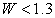m/s reduced R substantially and that is why is excluded from the consideration.

Figure 4 shows the dependence according to the air humidity at the experimental area on the air velocity and input water temperature.

The calculated value of the correlation coefficients is shown in the figure and is R = 0.92 and 0.98. As it can be seen from Figure 4 the results at the input water temperature of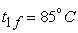have the relative humidity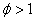which is explained by the experiment error.

Also, according to Figure 4, the increase of velocity reduces the relative humidity of air which is not able to become saturated with vapor. And the increase of input water temperature increases the evaporation and saturation efficiency. The data specified are processed in the further researches.

Also, according to Figure 4, the increase of velocity reduces the relative humidity of air which is not able to become saturated with vapor. And the increase of input water temperature increases the evaporation and saturation efficiency, relative deviation of points from the presumptive straight line does not exceed 25%. This data is processed in future.

### 4. Research Results of Polytropic Air Saturation and Heating with Water Cooling

The process considered is characterized by the gradients of temperature and pressure being directed air, which accelerates cooling of water in cooling towers.

As water temperature is higher than the air t7> t0 (t7 - t0) the latter is heated and due to the partial pressure gradient (p7 - p0), the air is saturated and water is decreased. The equation of heat balance in the cooling tower: the heat to the water = heat of the evaporation + heat received by the air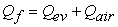(10)

Calculation of experimental heat transfer coefficient as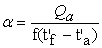(11)

where the heat received by the air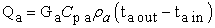(12)

In the equation (11) it is assumed that the middle air temperature near the water equals the temperature of water. And so instead of air temperature the average water temperature is taken.

To compare calculation of heat transfer coefficient in air, let’s theoretically. Assume the thickness of the heat layer in the air to equal thickness of boundary layer for air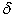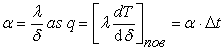(13)– the thickness of boundary layer for air from the Navier-Stokes equations.

The experimental and theoretical calculation of heat transfer coefficient in the air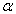, depending on the water temperature and the velocity of the air is shown in Figure 5. The upper curve is experimental and lower curve is theoretical calculation of heat transfer through the thickness of the layer displacement. It is seen that the boundary layer thickness at low air velocity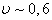m/s equals experimental results. But in the case of high velocity of air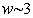m/s theoretical thickness is higher than experimental, as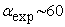, а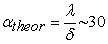Wt/K/m2.

Figure 5. Dependency of the heat transfer coefficient on the air velocity and the initial temperature of water (dots – experiment, lower curve – theory)

Figure 6 illustrates the heat of evaporation and heat received by the air depending on air velocity and initial water temperature

The heat of the evaporation, Wt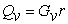(14)

The heat received by air is calculated by equation (12).

As shown in Figure 6, the heat of the evaporation greatly exceeds the one received by air in the full range of water temperature and air velocity. In addition, the growth of temperature by 35% (from 63 to 85C) increases the heat of evaporation twice and heat received by the air by 40% only.

The heat transfer coefficient in air is calculated by equation (11).

To calculate the heat transfer coefficient in the water film the thickness of displacement layer is determines theoretically.

Figure 6. Dependencies of specific heat spent on evaporation (two upper curves) and heat received by the air (two lower curves) on air velocity and initial temperature

At the water flow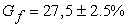kg/h and wetted perimeter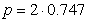m, as twice for corrugated netting packing, we have water concentration of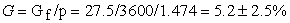g/m/s. Reynolds number for the water film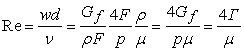(15)

As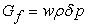, where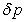– area of cross section and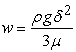≈ 5 s/sec – velocity of gravity flow of water film from Nusselt equation. The thickness of the film is calculated from equation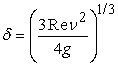(16)

For calculating heat transfer coefficient in water film, let us determine the film thickness (or half thickness), since the film is symmetric.

The experimental results are illustrated in Figure 7 with water concentration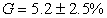g/(m c). Half of thickness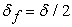depends on the temperature of input water as the water film thermal properties change and practically does not depend on the air velocity.

Half the thickness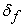of the water film was compared to the thickness of the displacement layer in it. Let us calculate thickness of the displacement layer theoretically .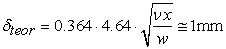(17)

As calculated, the thickness of the displacement layer of water film greatly exceeds the experimental thickness of the film. That is, the processes of heat transfer in the film take place entirely within the boundary layer and the transfer zone in the water film which equals half the thickness of the film.

Using the boundary layer density in water film let us calculate the heat transfer coefficient in the water film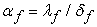.

Compare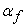to the heat transfer coefficient in the film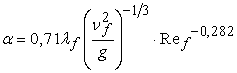(18)

where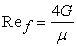,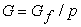,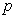– wetted perimeter.

Has shown in Figure 8 the heat transfer coefficient depends on the input temperature of the water and practically doesn’t depend the air velocity at constant water concentration as shown in Figure 5. The heat transfer coefficient increases with increase of input water temperature as change thermal properties of water film. The good deviation the known heat transfer coefficient in the film and the experimental results does not exceed 24% in the range of air velocities and an input water temperature.

Figure 8. The heat transfer coefficient in water film depending on the air velocity and the input water temperature (down two lines – calculation from )

Let us determine experimentally the difference of temperature at the center and edge of the water film from the heat balance (3)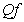, Wt.

According to 's heat law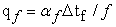(19)

where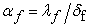the heat transfer coefficient calculated in the water film using half of the water film’s thickness.

Figure 9 illustrates the increase of the difference temperature at growth of the initial temperature of water film and air velocity.

The growth in is from 0.52 to 0.68 C (at input water film temperature 63C) and from 1.13 to 1.27C (at input water film temperature 85C) at water concentration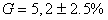g/m/s and at diapason of the air velocity 1.3…2.8 m/s.

Figure 9. The dependency of the difference of temperature in the water film on the air velocity and input water film temperature

The difference of temperature in the air flow is calculated experimentally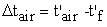, where the temperature of air at the edge of air flow equals the average temperature of water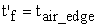. The Figure 10 illustrates the dependency of it on the air velocity and input water film temperature. The growth of air velocity from 1.3 to 2.8 m/s increases the temperature gradient normal to the air flow from 31.9 to 44.1C, or 44.2 to 54.3C at the input water film temperature 63, or 85C. That is much more than in the water film.

A comparison of the difference of temperature in Figure 9-10 illustrates that the difference in the water film is more dependent on air velocity and water film temperature as a result of influence of mass transfer on heat transfer.

Figure 10. Dependency of difference in the air temperature on the air velocity and input water temperature

### 5. Conclusions

The thickness of heat transfer dissipation layer is 3 times bigger than that of the boundary layer calculated theoretically.

The experimental thickness of the water film is much less than the thickness of the boundary layer in the water film calculated experimentally. Therefore, the process of heat transfer in the water film is unsteady.

The heat transfer in the water film with a deviation of up to 24 % is describes by a known equation.

The difference in water film temperature in the is about 0.52-1.28C and in the air 34.9-51.3C. This depends on input of water temperature and slightly depends on the air velocity.

### List of Abbreviations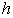, J/kg – enthalpy,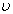, m/s – velocity,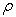, kg/m3 – density,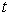, C – temperature,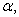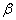– heat and mass transfer coefficients,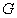, kg/s – flow rate,

с, J/kg/K – heat capacity,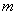, kg – mass,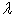, Wt/K/m2 – thermal conductivity,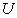, m/sec –water film velocity,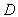m/sec– diffusion coefficient,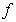, m2 – contact area,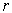, J/kg – latent heat,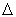–difference of input and output parameter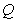, W, J/sec – heat,, m/s – air velocity,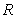– Pearson’s correlation coefficient,, m – displacement thickness,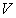, m3 – volume,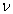, m2/s – viscosity,– relative humidity

Indexes:

s, w – humid air in stream and near the water film,

ls – loss,

v – vapor,

f – film,

in, out – input, output,

‘ – average.

### References

  Qureshi B. A., Zubair S. M. A complete model of wet cooling towers with fouling in ﬁlls. Applied Thermal Engineering. 2006. No. 26. P. 1982-1989.In article CrossRef  Naphon P. Study on the heat transfer characteristics of an evaporative cooling tower. International Communications in Heat and Mass Transfer. 2005. Vol. 32. P. 1066-1074.In article CrossRef  El'-Talla A. A. Teploobmin pry kontaktniy kondensatsiyi v trubakh: Ph.D. thesis in Engineering Science. Kyiv polytechnical institute, 1997. 220 p.In article  Berman L. D. Ysparytel'noe okhlazhdenye tsyrkulyatsyonnoy vody. Moskva - : HEY, 1957. 424 p.In article  Alekseev V. P. Yssledovanye protsessov teplo- y massoobmena v apparatakh kholodyl'nikh ustanovok s rehulyarnimy nasadkamy.: Ph.D. thesis in Engineering Science. Odesa, 1969. 246 p.In article  Heorhalina O. R. Modelyuvannya ta optymizatsiya plivkovykh okholodzhuvachiv Ph.D. thesis in Engineering Science. Kyiv polytechnical institute. Odesa., 2004. 185 p.In article  Heshev P.Y., Kovalev O.P., Tsvelodub O.Yu., Yakubovskyy Yu.V Teplomassoobmen pry kontakte horyacheho haza so stekayushchey plenkoy zhydkosty. YFZh, 1984. - t. 46. - # 3. P. 428-432.In article  Tuz V.E. Kontaktniy teplomassoobmenniy apparat s porystoy nasadkoy dlya toplyvnoy systemi promishlennoy gas turbine Ph.D. thesis in Engineering Science. Kyiv polytechnical institute, 1989. 200 p.In article  Petruchyk A.Y., Fysenko S.P. Matematycheskoe modelyrovanye ysparytel'noho okhlazhdenyya plenok vodi v hradyrnyakh. YFZh. 1999. - t. 72. - #1. P. 43-49.In article  Antonyk V.V., Petruchyk A.Y., Solodukhyn A.D., Stolovych N.N., Fysenko S.P. Teplomassoobmen pry ysparytel'nom okhlazhdenyy plenok vodi na dvukh vertykal'nikh plastynakh. Trudi IV Mynskoho Mezhdunarodnoho foruma po teplomassoobmenu. - 2000- P. 314-325.In article  Chou Y., Ruey-Jen Y. The evaporation of a saturated porous layer inside an inclined airflow channel. International Journal of Heat and Fluid Flow. 2007, No. 28, P. 407-417.In article CrossRef  Saouli S., Boumaza M., Settou N., Aiboud-Saoili S., Daguenet M. Numerical study of the evaporation of a falling Ostwaldian film along an inclined flat plate into a laminar stream of humid air. 4th International Conference on heat Transfer, Fluid Mechanics and Thermodynamics (HEFAT 2005), . .In article  Feddaoui M., Meftah H., Mir A. The numerical computation of the evaporative cooling of falling water film in turbulent mixed convection inside a vertical tube. International Communications in Heat and Mass Transfer. 2006. No. 33. P. 917-927.In article CrossRef  Zhang H., Tao W., He Y., Zhang W. Numerical study of liquid film cooling in a rocket combustion chamber. International Journal of Heat and Mass Transfer. 2006. No. 49. P. 349-358.In article CrossRef  Kuzmenko I. M. Hidrodynamika ta teplomasoobmin v protytokovomu kontaktnomu vyparovuvachi z sitchastoyu hofrovanoyu nasadkoyu Ph.D. thesis in Engineering Science. Kyiv polytechnical institute, 2003-200 p.In article  H. Schlichting, BoundaryLayer Theory, McGraw-Hill (1960) 510 p.In article